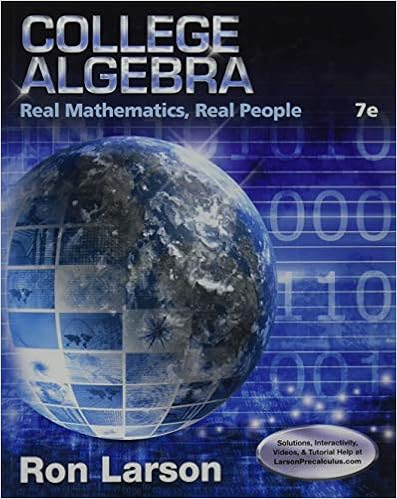# 05 Matrix Algebra (Notes).pdf - 525 6.3 Matrix Algebra Andy...

• sukkhdev55555
• 15

This preview shows page 1 - 4 out of 15 pages.

##### We have textbook solutions for you!
The document you are viewing contains questions related to this textbook.The document you are viewing contains questions related to this textbook.
Chapter 5 / Exercise 68
College Algebra: Real Mathematics, Real People
LarsonExpert Verified
6.3 Matrix Algebra 525 6.3 Matrix Algebra Objectives 1. Add and Subtract Matrices 2. Multiply a Matrix by a Constant 3. Multiply Matrices 4. Solve Problems Using Matrices 5. Recognize the Identity Matrix With certain restrictions, we can add, subtract, and multiply matrices. In fact, many problems can be solved using the arithmetic of matrices. To illustrate the addition of matrices, suppose there are 108 police officers employed at two different locations: Andy Nelson/The Christian Science Monitor via Getty Images Downtown Station Male Female Day shift 21 18 Night shift 12 6 Suburban Station Male Female Day shift 14 12 Night shift 15 10 The employment information about the police officers is contained in two matrices. and The entry 21 in matrix indicates that 21 male officers work the day shift at the downtown station. The entry 10 in matrix indicates that 10 female officers work the night shift at the suburban station. To find the city-wide totals, we can add the corresponding entries of matrices and : D S c 21 18 12 6 d c 14 12 15 10 d c 35 30 27 16 d S D S D S c 14 12 15 10 d D c 21 18 12 6 d We interpret the total to mean: Male Female 35 30 27 16 Day shift Night shift
##### We have textbook solutions for you!
The document you are viewing contains questions related to this textbook.The document you are viewing contains questions related to this textbook.
Chapter 5 / Exercise 68
College Algebra: Real Mathematics, Real People
LarsonExpert Verified
To illustrate how to multiply a matrix by a number, suppose that one-third of the officers at the downtown station retire. The downtown staff then would consist of officers. We can compute by multiplying each entry of matrix by . 2 3 D 2 3 c 21 18 12 6 d c 14 12 8 4 d 2 3 D 2 3 D 2 3 D 526 Chapter 6 Linear Systems After retirements, the downtown staff will be Male Female 14 12 8 4 Day shift Night shift These examples illustrate two calculations used in the algebra of matrices, which is the topic of this section. We begin by giving a formal definition of a matrix and defining when two matrices are equal. An matrix is a rectangular array of numbers arranged in rows and columns. We say that the matrix is of size (or order ) . Matrices often are denoted by letters such as , , and . To denote the entries in an matrix , we use double-subscript notation: The entry in the first row, third column is , and the entry in the th row, th column is . We can use any of the following notations to denote the matrix : , , rows columns Two matrices are equal if they are the same size, with the same entries in cor- responding positions. If and are both matrices, then provided that each entry in matrix is equal to the corresponding entry in matrix . The following matrices are equal, because they are the same size and corre- sponding entries are equal. The following matrices are not equal, because they are not the same size. The first matrix is , and the second is . 1. Add and Subtract Matrices We can add matrices of the same size by adding the entries in corresponding positions. 3 3 2 3 c 1 2 3 1 2 3 d £ 1 2 3 1 2 3 1 2 3 § c 2 9 0.5 1 4 d c 3 1 2 1 2 2 d B b ij A a ij A B m n B [ b ij ] A [ a ij ] Equality of Matrices n m a 11 a 12 a 13 p a 1 n a 21 a 22 a 23 p a 2 n o o o o a m 1 a m 2 a m 3 p a mn ¥ [ a ij ] A A m n a ij j i a 13 A m n C B A m n n m mn m n Matrices
Let and be two matrices. The sum, , is the matrix
•••